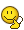# Convert Julian Dates to Decimal

## Homework Statement

There's an example problem in Astrophysics textbook that involves calculating T (time elapsed since last reference date as a decimal)
the date is July 30, 2005 at noon. and the reference date is Jan 01, 2000 at noon. the calendar starts on Jan 01, 2000 so that it is just 2000

the example problem gave the date as 2005.575

## Homework Equations

the formula is T = (t - JD2000) / 100 where t is today's date expressed as a decimal

## The Attempt at a Solution

I have tried taking July 30 as the 211 day in the year and divided it by 365.25. this gave me .578
I have tried adding 1.25 days to 211 (quarter day for 2000, 2001, 2002, 2003, and 2004) and dividing that by 365.25. ===.5811
I have tried adding 1.25 + the fraction of .25 up until 211 days for 2005 and got .5815.

I have tried a variety of things. I worked backwards from .575 and found that in order to get this figure, I have to divide 211 days by 367.

Maybe the textbook just rounded weird. or maybe there is some formula I'm supposed to be using to calculate this correctly. and if I don't have that formula, I will make fatal errors in later calculations.

phyzguy
•D'OH!